# Backtracking - Explanation and N queens problem

Oct. 21, 2017 170670

## What is backtracking?

Backtracking is finding the solution of a problem whereby the solution depends on the previous steps taken. For example, in a maze problem, the solution depends on all the steps you take one-by-one. If any of those steps is wrong, then it will not lead us to the solution. In a maze problem, we first choose a path and continue moving along it. But once we understand that the particular path is incorrect, then we just come back and change it. This is what backtracking basically is.

In backtracking, we first take a step and then we see if this step taken is correct or not i.e., whether it will give a correct answer or not. And if it doesn’t, then we just come back and change our first step. In general, this is accomplished by recursion. Thus, in backtracking, we first start with a partial sub-solution of the problem (which may or may not lead us to the solution) and then check if we can proceed further with this sub-solution or not. If not, then we just come back and change it.

Thus, the general steps of backtracking are:

• check if this sub-solution will lead to the solution or not
• If not, then come back and change the sub-solution and continue again

## N queens on NxN chessboard

One of the most common examples of the backtracking is to arrange N queens on an NxN chessboard such that no queen can strike down any other queen. A queen can attack horizontally, vertically, or diagonally. The solution to this problem is also attempted in a similar way. We first place the first queen anywhere arbitrarily and then place the next queen in any of the safe places. We continue this process until the number of unplaced queens becomes zero (a solution is found) or no safe place is left. If no safe place is left, then we change the position of the previously placed queen.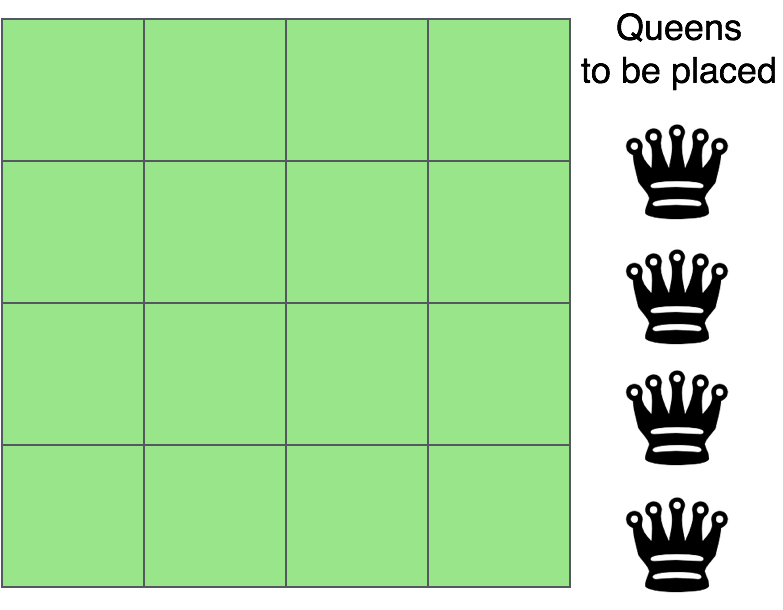The above picture shows an NxN chessboard and we have to place N queens on it. So, we will start by placing the first queen.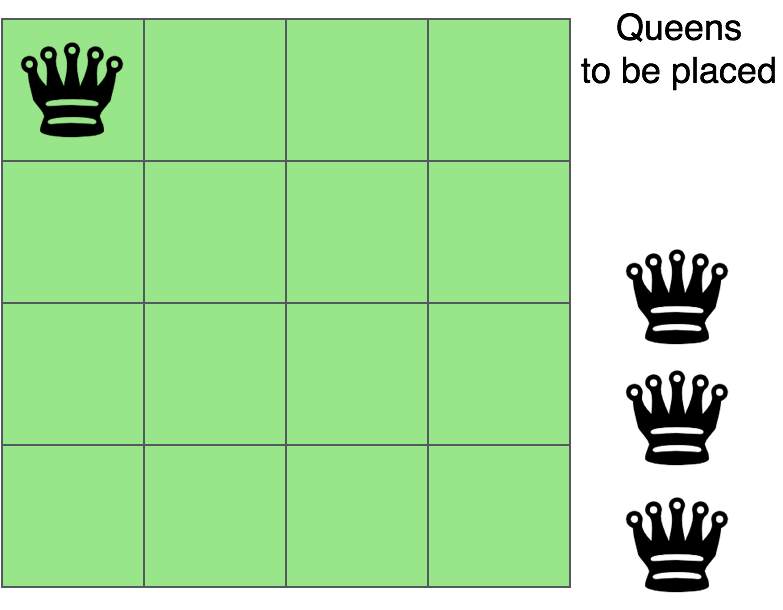Now, the second step is to place the second queen in a safe position and then the third queen.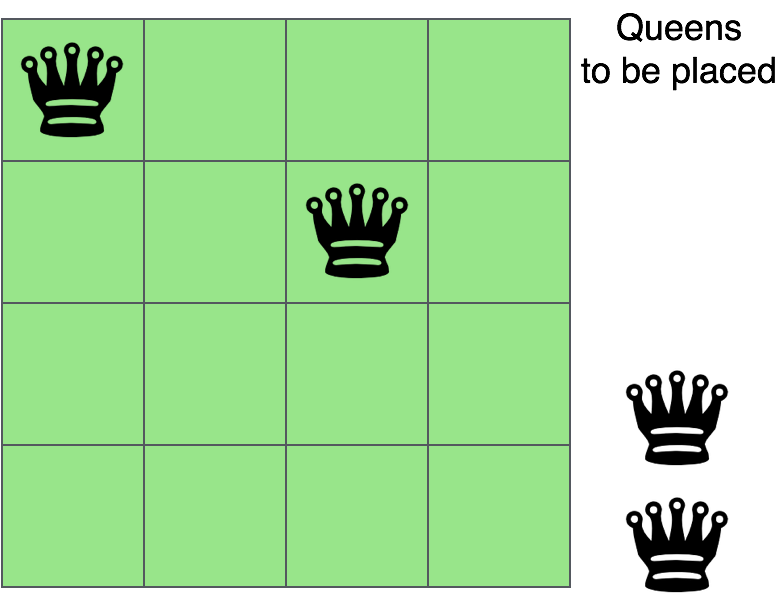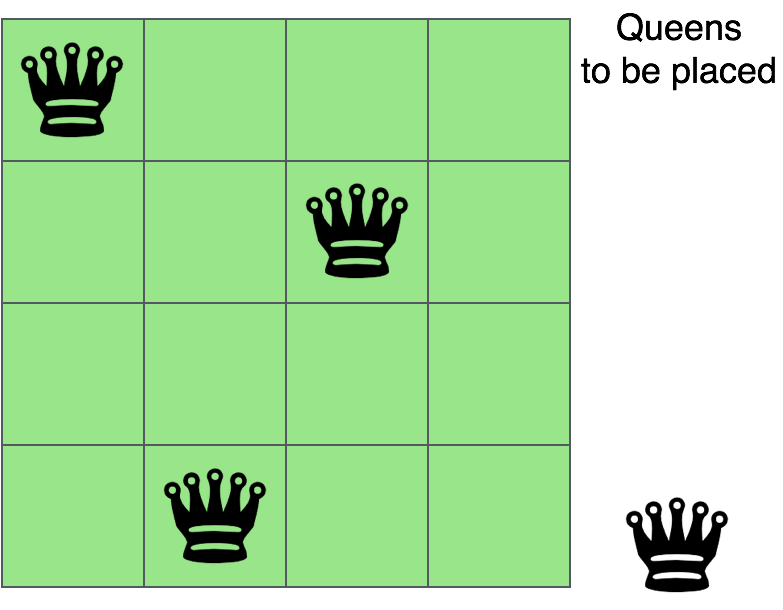Now, you can see that there is no safe place where we can put the last queen. So, we will just change the position of the previous queen. And this is backtracking.

Also, there is no other position where we can place the third queen so we will go back one more step and change the position of the second queen.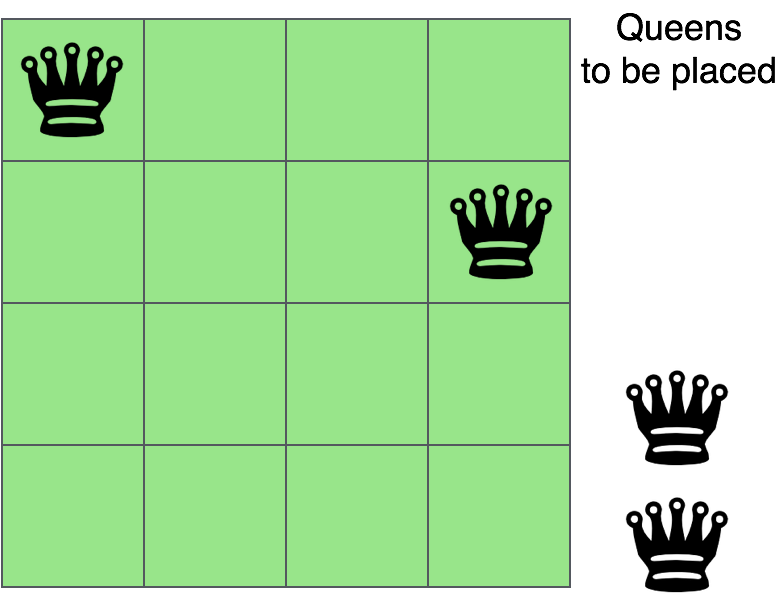And now we will place the third queen again in a safe position until we find a solution.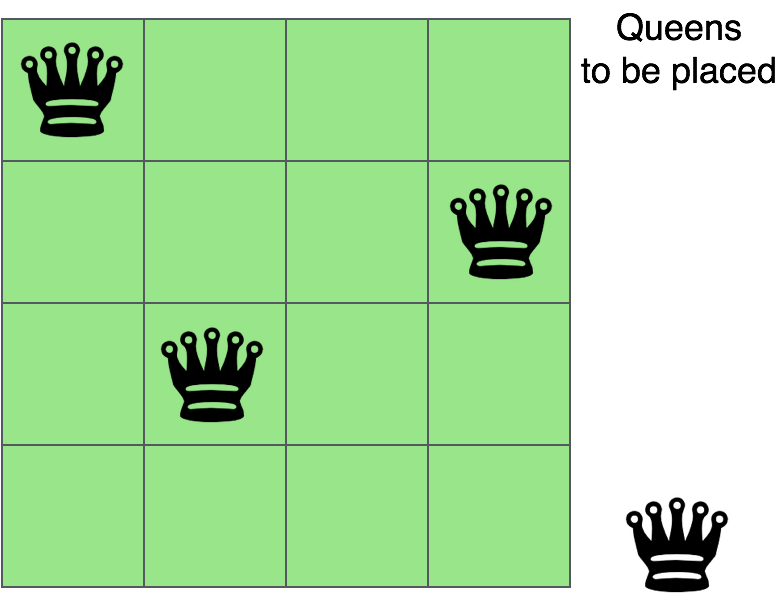We will continue this process and finally, we will get the solution as shown below.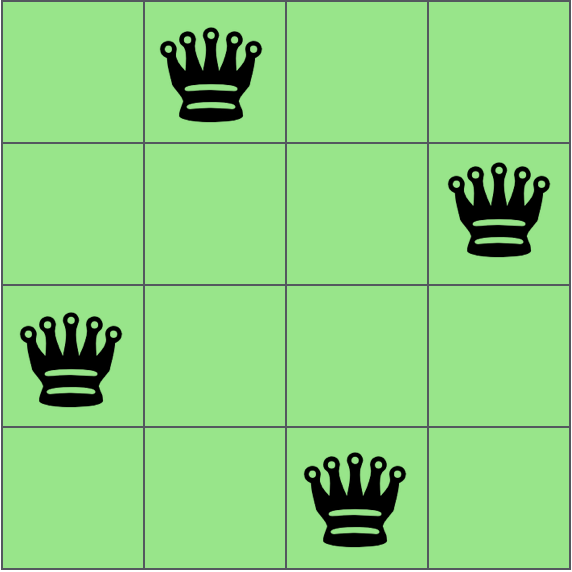As now you have understood backtracking, let us now code the above problem of placing N queens on an NxN chessboard using the backtracking method.

### C

#include <stdio.h>

//Number of queens
int N;

//chessboard
int board;

//function to check if the cell is attacked or not
int is_attack(int i,int j)
{
int k,l;
//checking if there is a queen in row or column
for(k=0;k<N;k++)
{
if((board[i][k] == 1) || (board[k][j] == 1))
return 1;
}
//checking for diagonals
for(k=0;k<N;k++)
{
for(l=0;l<N;l++)
{
if(((k+l) == (i+j)) || ((k-l) == (i-j)))
{
if(board[k][l] == 1)
return 1;
}
}
}
return 0;
}

int N_queen(int n)
{
int i,j;
//if n is 0, solution found
if(n==0)
return 1;
for(i=0;i<N;i++)
{
for(j=0;j<N;j++)
{
//checking if we can place a queen here or not
//queen will not be placed if the place is being attacked
if((!is_attack(i,j)) && (board[i][j]!=1))
{
board[i][j] = 1;
//recursion
//wether we can put the next queen with this arrangment or not
if(N_queen(n-1)==1)
{
return 1;
}
board[i][j] = 0;
}
}
}
return 0;
}

int main()
{
//taking the value of N
printf("Enter the value of N for NxN chessboard\n");
scanf("%d",&N);

int i,j;
//setting all elements to 0
for(i=0;i<N;i++)
{
for(j=0;j<N;j++)
{
board[i][j]=0;
}
}
//calling the function
N_queen(N);
//printing the matix
for(i=0;i<N;i++)
{
for(j=0;j<N;j++)
printf("%d\t",board[i][j]);
printf("\n");
}

}


### Java

import java.util.*;

class Queen
{
//number of queens
private static int N;
//chessboard
private static int[][] board = new int;

//function to check if the cell is attacked or not
private static boolean isAttack(int i,int j)
{
int k,l;
//checking if there is a queen in row or column
for(k=0;k<N;k++)
{
if((board[i][k] == 1) || (board[k][j] == 1))
return true;
}
//checking for diagonals
for(k=0;k<N;k++)
{
for(l=0;l<N;l++)
{
if(((k+l) == (i+j)) || ((k-l) == (i-j)))
{
if(board[k][l] == 1)
return true;
}
}
}
return false;
}

private static boolean nQueen(int n)
{
int i,j;
//if n is 0, solution found
if(n==0)
return true;
for(i=0;i<N;i++)
{
for(j=0;j<N;j++)
{
//checking if we can place a queen here or not
//queen will not be placed if the place is being attacked
if((!isAttack(i,j)) && (board[i][j]!=1))
{
board[i][j] = 1;
//recursion
//wether we can put the next queen with this arrangment or not
if(nQueen(n-1)==true)
{
return true;
}
board[i][j] = 0;
}
}
}
return false;
}

public static void main(String[] args)
{
Scanner s = new Scanner(System.in);
//taking the value of N
System.out.println("Enter the value of N for NxN chessboard");
N = s.nextInt();

int i,j;
//calling the function
nQueen(N);
//printing the matix
for(i=0;i<N;i++)
{
for(j=0;j<N;j++)
System.out.print(board[i][j]+"\t");
System.out.print("\n");
}

}
}


### Python

#Number of queens
print ("Enter the number of queens")
N = int(input())

#chessboard
#NxN matrix with all elements 0
board = [*N for _ in range(N)]

def is_attack(i, j):
#checking if there is a queen in row or column
for k in range(0,N):
if board[i][k]==1 or board[k][j]==1:
return True
#checking diagonals
for k in range(0,N):
for l in range(0,N):
if (k+l==i+j) or (k-l==i-j):
if board[k][l]==1:
return True
return False

def N_queen(n):
#if n is 0, solution found
if n==0:
return True
for i in range(0,N):
for j in range(0,N):
'''checking if we can place a queen here or not
queen will not be placed if the place is being attacked
if (not(is_attack(i,j))) and (board[i][j]!=1):
board[i][j] = 1
#recursion
#wether we can put the next queen with this arrangment or not
if N_queen(n-1)==True:
return True
board[i][j] = 0

return False

N_queen(N)
for i in board:
print (i)


## Explanation of the code

is_attack(int i,int j) →  This is a function to check if the cell (i,j) is under attack by any other queen or not. We are just checking if there is any other queen in the row ‘i’ or column ‘j’. Then we are checking if there is any queen on the diagonal cells of the cell (i,j) or not. Any cell (k,l) will be diagonal to the cell (i,j) if k+l is equal to i+j or k-l is equal to i-j.

N_queen → This is the function where we are really implementing the backtracking algorithm.

if(n==0) → If there is no queen left, it means all queens are placed and we have got a solution.

if((!is_attack(i,j)) && (board[i][j]!=1)) → We are just checking if the cell is available to place a queen or not. is_attack function will check if the cell is under attack by any other queen and board[i][j]!=1 is making sure that the cell is vacant. If these conditions are met then we can put a queen in the cell – board[i][j] = 1.

if(N_queen(n-1)==1) → Now, we are calling the function again to place the remaining queens and this is where we are doing backtracking. If this function (for placing the remaining queen) is not true, then we are just changing our current move – board[i][j] = 0 and the loop will place the queen on some another position this time.

I will also discuss more problems on this topic in the upcoming posts.

Liked the post?
Developer and founder of CodesDope.